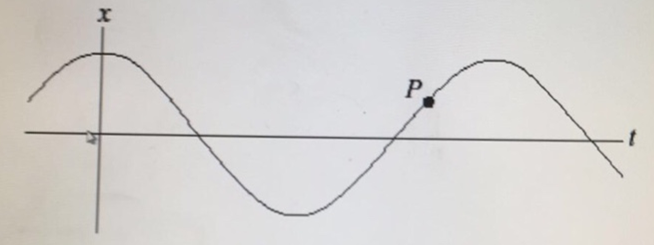# Problem: A mass attached to a spring oscillator back and forth as indicated in the position vs. time plot below.  At point P,1. the x-component of the velocity is negative and the x-component of the acceleration is positive.2.  the x-component of the velocity is negative and the x-component of the acceleration is negative.3. the x-component of the velocity is zero and the x-component of the acceleration is zero.4. the x-component of the velocity is positive and the x-component of the acceleration is positive.5. the x-component of the velocity is positive and the x-component of the acceleration is negative.

###### FREE Expert Solution

Position vs. time curve presented in an equation form shows that mass is moving towards positive amplitude at P.

Its velocity would be positive and the force on it is in the opposite direction. That is in the negative x-axis.

80% (292 ratings)###### Problem Details

A mass attached to a spring oscillator back and forth as indicated in the position vs. time plot below.  At point P,1. the x-component of the velocity is negative and the x-component of the acceleration is positive.

2.  the x-component of the velocity is negative and the x-component of the acceleration is negative.

3. the x-component of the velocity is zero and the x-component of the acceleration is zero.

4. the x-component of the velocity is positive and the x-component of the acceleration is positive.

5. the x-component of the velocity is positive and the x-component of the acceleration is negative.